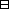## Group Theory and Linear Algebra

Last updated: 23 September 2014

## Lecture 19: Polar decomposition

Let $f:V\to V$ be a linear transformation. Let $⟨,⟩:V×V\to ℂ$ be a positive definite Hermitian form. Show that the following are equivalent.

 (a) $f$ is self adjoint and all eigenvalues are positive. (b) There exists $g:V\to V$ such that $g$ is self adjoint and $f={g}^{2}\text{.}$ (c) There exists $h:V\to V$ such that $f=h{h}^{*}\text{.}$ (d) $f$ is self adjoint and $⟨f\left(v\right),v⟩\ge 0$ for all $v\in V\text{.}$Proof.

To show: (a) $⇒$ (b) $⇒$ (c) $⇒$ (d) $⇒$ (a).

(a) $⇒$ (b) Assume $f$ is self adjoint and all eigenvalues are positive.
To show: There exists $g:V\to V$ such that $g$ is self adjoint and $f={g}^{2}\text{.}$
Since $f$ is self adjoint, $f$ is normal.
By the spectral theorem, there exists an orthonormal basis $B=\left\{{b}_{1},{b}_{2},\dots ,{b}_{k}\right\}$ such that $Bf= ( d10 d2 ⋱ 0dk ) .$ Since all eigenvalues of $f$ are positive, ${d}_{1},{d}_{2},\dots ,{d}_{k}\in {ℝ}_{\ge 0}\text{.}$
Let $Bg= ( d10 ⋱ 0dk )$ be the matrix of $g:V\to V\text{.}$

To show:
 (1) $g$ is self adjoint. (2) $f={g}^{2}\text{.}$
 (1) To show: $g={g}^{*}\text{.}$ $Bg*= Bg‾t= ( d1‾0 ⋱ 0dk‾ ) = ( d10 ⋱ 0dk ) =Bg,$ since $\sqrt{{d}_{1}},\dots ,\sqrt{{d}_{k}}\in ℝ\text{.}$ So ${g}^{*}=g\text{.}$ (2) To show: $f={g}^{2}\text{.}$ $Bg2= (Bg)2= ( d10 ⋱ 0dk ) 2 = ( d10 ⋱ 0dk ) =Bf.$ So ${g}^{2}=f\text{.}$

(b) $⇒$ (c) Assume there exists $g:V\to V$ such that $g$ is self adjoint and $f={g}^{2}\text{.}$
To show: There exists $h:V\to V$ such that $f=h{h}^{*}\text{.}$
Let $h=g\text{.}$
To show: $f=h{h}^{*}\text{.}$ $hh*=gg*=gg =g2=f.$ $\text{(}g={g}^{*}$ since $f$ is self adjoint.)

(c) $⇒$ (d) Assume there exists $h:V\to V$ such that $f=h{h}^{*}\text{.}$

To show:
 (a) $f$ is self adjoint. (b) If $v\in V$ then $⟨f\left(v\right),v⟩\ge 0\text{.}$
 (1) To show: $f={f}^{*}\text{.}$ $f* = (hh*)*= (h*)*h*, (ab)*= b*a*, = hh*=f,since (a*)*=a.$ (2) Assume $v\in V\text{.}$ To show: $⟨f\left(v\right),v⟩\in {ℝ}_{\ge 0}\text{.}$ $⟨f(v),v⟩= ⟨hh*v,v⟩= ⟨h*v,h*v⟩ ∈ℝ≥0,$ since $⟨,⟩$ is positive definite.

(d) $⇒$ (a) Assume $f$ is self adjoint and if $v\in V$ then $⟨f\left(b\right),v⟩\in {ℝ}_{\ge 0}\text{.}$

To show:
 (1) $f$ is self adjoint. (2) All eigenvalues of $f$ are positive.
 (1) To show: $f$ is self adjoint. By assumption, $f$ is self adjoint. (2) To show: All eigenvalues of $f$ are positive. To show: If $\lambda \in ℂ$ and $v\in V$ and $fv=\lambda v$ then $\lambda \in {ℝ}_{\ge 0}\text{.}$ Assume $\lambda \in ℂ$ and $v\in V$ and $fv=\lambda v\text{.}$ To show: $\lambda \in {ℝ}_{\ge 0}\text{.}$ We know: $⟨f\left(v\right),v⟩\in {ℝ}_{\ge 0}\text{.}$ So $⟨fv,v⟩= ⟨λv,v⟩= λ⟨v,v⟩ ∈ℝ≥0.$ Since $⟨v,v⟩\in {ℝ}_{\ge 0},$ because $⟨,⟩$ is positive definite, then $\lambda \in {ℝ}_{\ge 0}\text{.}$

$\square$

Let $A\in {GL}_{n}\left(ℂ\right)\text{.}$ Then there exist $P,$ diagonalisable with positive eigenvalues, and $U,$ unitary, such that $A=PU.$Idea of proof. Let $P$ be such that ${P}^{2}=A{\stackrel{‾}{A}}^{t},$ and $U={P}^{-1}A\text{.}$ $\square$

## Notes and References

These are a typed copy of Lecture 19 from a series of handwritten lecture notes for the class Group Theory and Linear Algebra given on September 6, 2011.